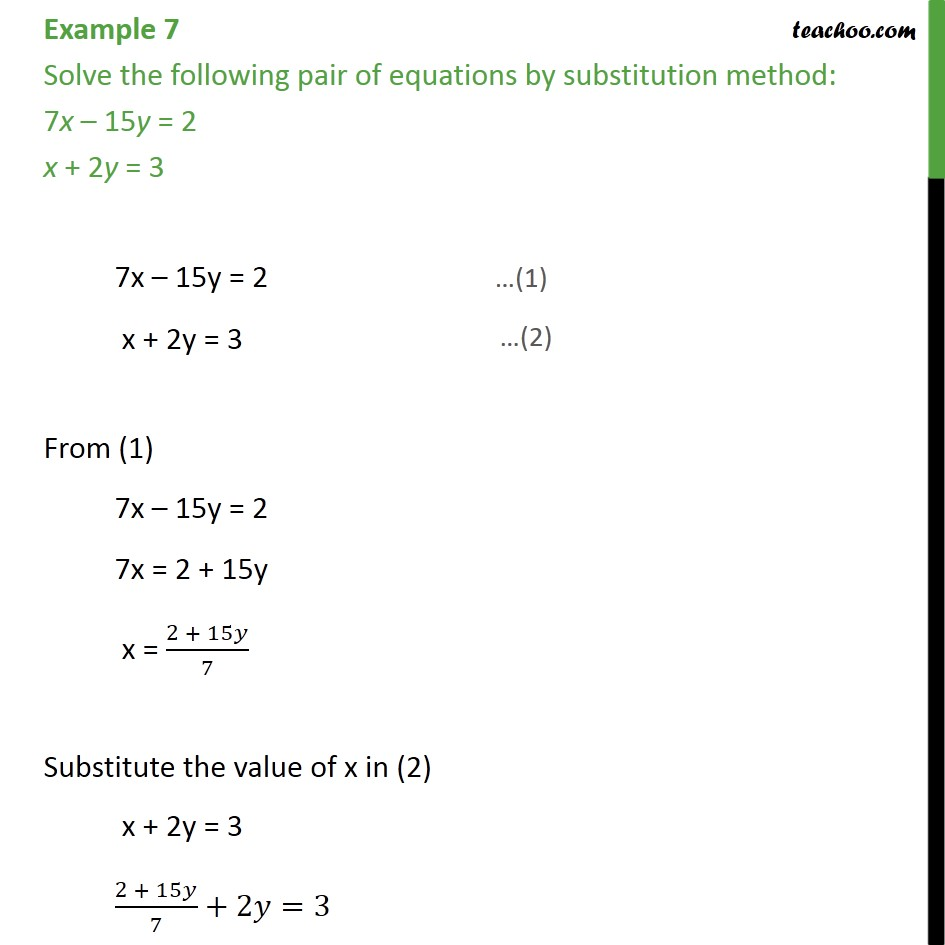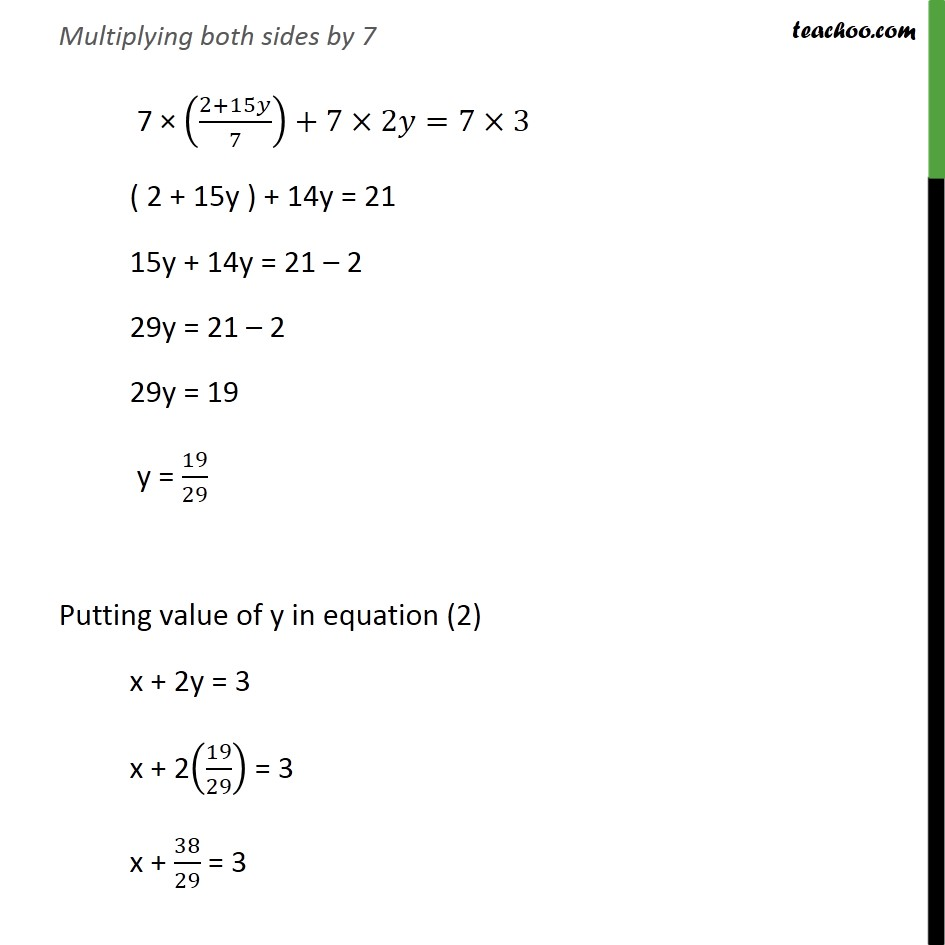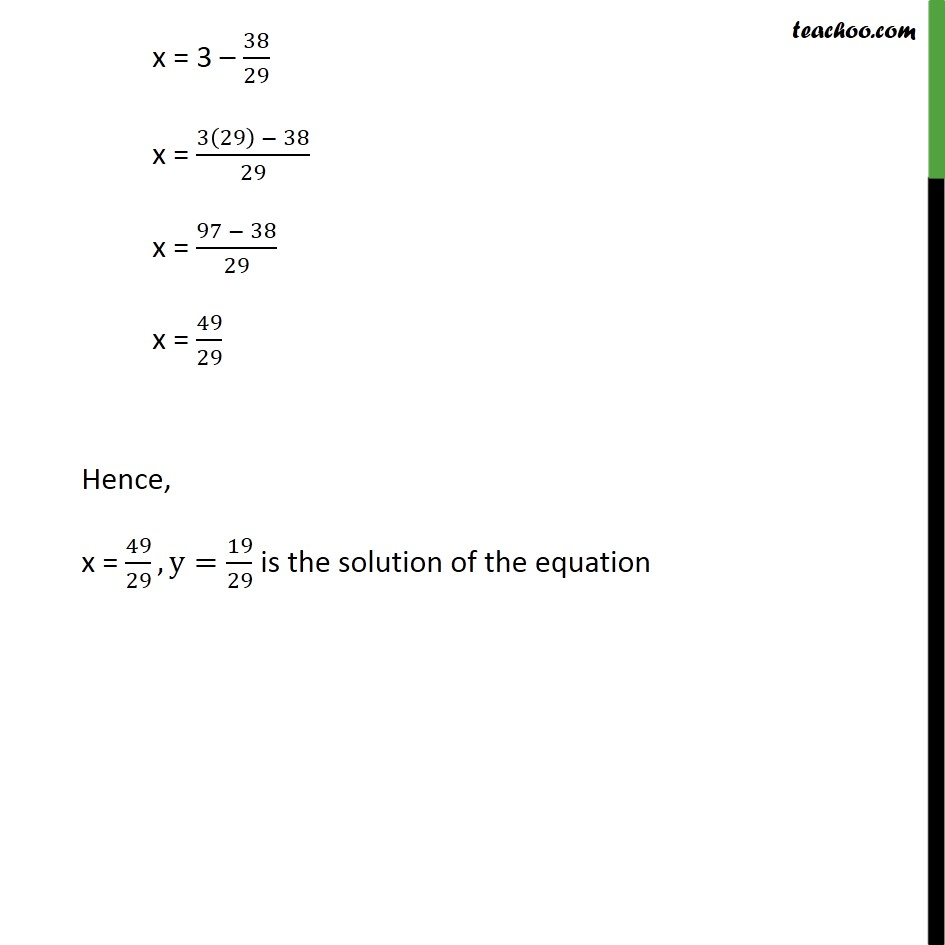1. Chapter 3 Class 10 Pair of Linear Equations in Two Variables
2. Concept wise
3. Substitution

Transcript

Example 7 Solve the following pair of equations by substitution method: 7x – 15y = 2 x + 2y = 3 7x – 15y = 2 x + 2y = 3 From (1) 7x – 15y = 2 7x = 2 + 15y x = (2 + 15𝑦)/7 Substitute the value of x in (2) x + 2y = 3 (2 + 15𝑦)/7+2𝑦=3 Multiplying both sides by 7 7 × ((2+15𝑦)/7)+7×2𝑦=7×3 ( 2 + 15y ) + 14y = 21 15y + 14y = 21 – 2 29y = 21 – 2 29y = 19 y = 19/29 Putting value of y in equation (2) x + 2y = 3 x + 2(19/29) = 3 x + 38/29 = 3 x = 3 – 38/29 x = (3(29) − 38)/29 x = (97 − 38)/29 x = 49/29 Hence, x = 49/29,y=19/29 is the solution of the equation

Substitution